# Hands-on Digital transmission with your soundcard¶

## 03 - Baseband signal transmission¶

In the previous notebook we introduced a streaming object for simulating the audio channel. In this notebook, we will generate a baseband signal and observe the received signal from the audio channel and simulated channels.

We will use the linear real-valued modulation given by

$$x(t) = \sum_{m=-\infty}^{\infty} d[m]g(t-mT)$$

where $d[m]$ is the BPSK-modulated bitstream, i.e. $$d[m] = \begin{cases} 1 & b[m] = 0 \\ -1 & b[m] = 1\end{cases}$$ and $b[m]$ is the bit to be transmitted at the $m$th symbol. Moreover, $g(t)$ is the transmit filter and $T$ is the symbol rate. For more detailed description of above equations, refer for example to DSPIllustrations.com - The First Nyquist Criterion and DSPIllustrations.com - Eye Diagram Examples.

First, let's get the standard stuff imported:

In :
import matplotlib
import matplotlib.pyplot as plt
%matplotlib notebook

import numpy as np
import sys; sys.path.append('..')

In :
try:
except ModuleNotFoundError:
print ("Did not find tikzmagic. You will not be able to compile the tex code!")

In :
%matplotlib notebook

In :
from audioComms import Environment
from audioComms.plotting import PlotSpectrum, PlotWaveform


First, let's define some filters:

In :
# Source code has been redacted in online version
# Omitting 16 lines of source code


Try out these filters by plotting them:

In :
t = np.linspace(-4,4,1000)
plt.figure(figsize=(8,3))
plt.plot(t, get_filter('rc', 1, rolloff=0.5)(t), label=r'RC $\alpha=0.5$')
plt.plot(t, get_filter('rc', 1, rolloff=0.9)(t), label=r'RC $\alpha=0.9$')
plt.plot(t, get_filter('rect', 1)(t), label='Rect');

plt.grid(True); plt.xlabel('t [s]'); plt.ylabel('g(t)'); plt.legend();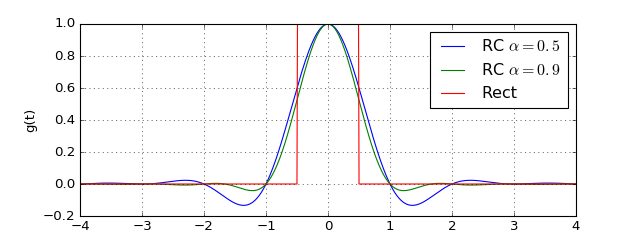Now, let's define a transmitter that generates the according baseband signal. Here, we make use of the library function StatefulFilter which implements a linear filter which keeps the state between subsequent calls to its filter function:

In :
from audioComms import TX
from audioComms.utils import StatefulFilter

In :
# Source code has been redacted in online version
# Omitting 19 lines of source code


Let's try out this signal generator:

In :
samplerate=44100
env = Environment(samplerate=samplerate)
Ts = 1/441  # symbol duration
t = np.arange(-4*Ts, Ts, 1/samplerate)

T = TransmitSignal(env, get_filter('rc', Ts, rolloff=0.5)(t), Ts)
plt.figure(figsize=(8,3))
x = T._generateSignal()
t = np.arange(len(x)) / (samplerate * Ts)
plt.plot(t, T._generateSignal());
plt.grid(True); plt.xlabel('t/Ts'); plt.ylabel('x(t)'); plt.tight_layout();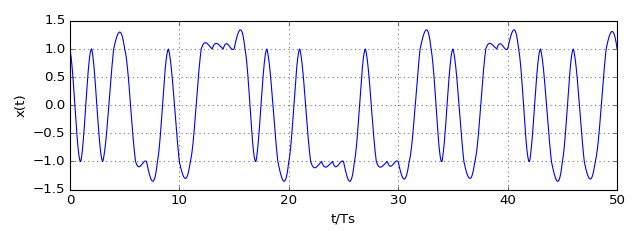Now, let's transmit this signal through the channel and plot the eye diagram. To plot the diagram, we use the library object PlotEyeDiagram. In a block diagram, the chain looks as follows:

In :
%%tikz -l positioning, --size=800,240
\tikzset{block/.style={draw,thick,minimum width=2cm,minimum height=1cm}}
\tikzset{new/.style={fill=red!50!white}}

\node (T) [block,new]  {TransmitSignal};
\node (C) [block,right=of T] {Channel};
\node (E) [block,right=of C,new] {Plot Eye diagram};

\draw [-stealth] (T) -- (C);
\draw [-stealth] (C) -- (E);In :
from audioComms.channels import AudioChannel, SimulatedChannel, IdentityChannelEffect
from audioComms.plotting import PlotEyeDiagram

In :
# Source code has been redacted in online version
# Omitting 14 lines of source code

In :
runTransmission('rc', lambda env: SimulatedChannel(environment=env, channelEffect=IdentityChannelEffect(gain=0.2)))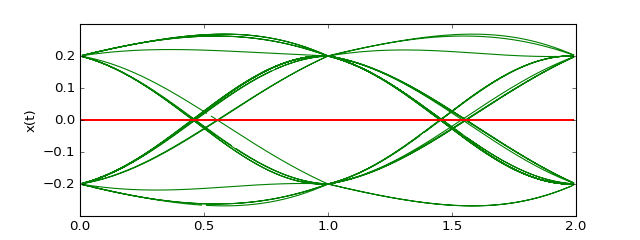Stop received from external


Apparently, we see a perfect eye diagram with the simulated channel. Now, let's try this with the real audio channel:

In :
runTransmission('rc', lambda env: AudioChannel(env))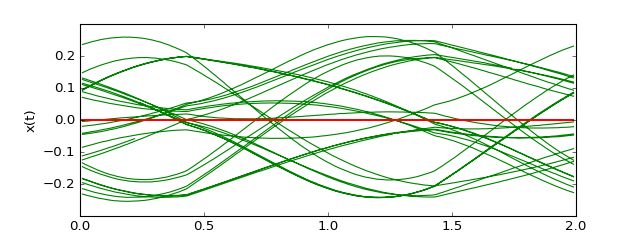Stop received from external


Unfortunately, the eye pattern is not visible at all. How can that be? Didn't we see in the first notebook that the sound device accurately transmits the signal? Let's make some additional test, by using a rectangular filter for the pulse shaping. First, we run it in the simulated channel:

In :
runTransmission('rect', lambda env: SimulatedChannel(env,channelEffect=IdentityChannelEffect(gain=0.2)))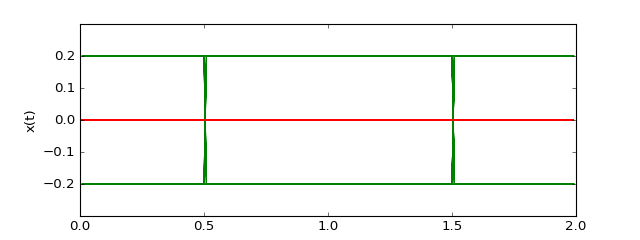Stop received from external


The simulated channel shows the perfect eye diagram. Let's compare against the eye diagram from the audio channel:

In :
runTransmission('rect', lambda env: AudioChannel(env))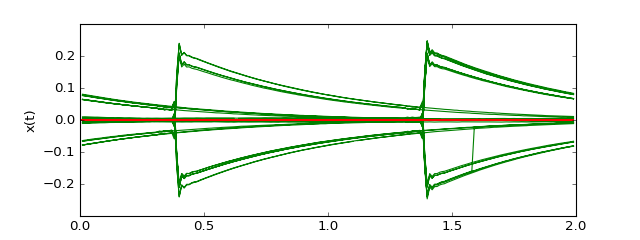Stop received from external


Uh, oh, what's happening there? Where are the rects gone? Instead, it more looks like a bunch of RC discharging curves. How can that be? Remember, the RC circuit acts as a lowpass. Also, what's usually written in the frequency range of audio devices? Something like 20-20000Hz. Ah, the sondcard acts like a highpass for our signal, maybe with cutoff 20Hz. Let's try to replicate this behaviour with a simulation. We just add a highpass to the channel output (with RC Transfer function):

In :
from audioComms.channels import HighpassChannelEffect
runTransmission('rect', lambda env: SimulatedChannel(env, channelEffect=HighpassChannelEffect(gain=0.1)))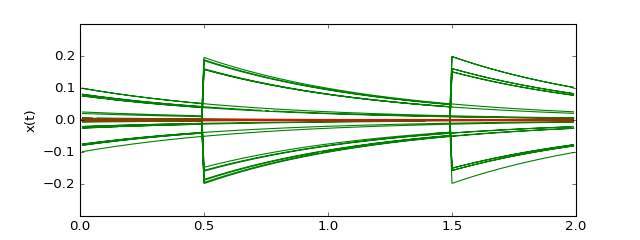Stop received from external


Suddenly, the simulated eye diagram nicely matches the audio channel. Note, that depending on your audio device, the filter would need to be adapted to match the audio channel.

Apparently, our signals all have significant contribution in the low frequency region. Hence, they are not suitable for the baseband transmission with the soundcard, as we do not get a clear eye diagram. How can this issue be resolved? There are some possibilties:

Before we actually overcome the problem of the highpass character of the audio device, let's measure the audio behaviour around DC. We create a transmitter that transmits pure Gaussian noise samples. At the receiver we plot the spectrum:

In :
class TransmitNoise(TX):
def __init__(self, environment):
super().__init__(environment)

def _generateSignal(self):
return np.random.randn(5000)

def runNoiseTransmission(channelFunc):
samplerate=44100
env = Environment(samplerate=samplerate)

transmitter = TransmitNoise(env)
channel = channelFunc(env)#SimulatedChannel(environment=env, channelEffect=IdentityChannelEffect(gain=0.2))
plotSpec = PlotSpectrum(env, windowDuration=2, logScale=True, figsize=(8,3), xlim=(-1000, 1000), ylim=(-100, -40))

transmitter.transmitsTo(channel)
channel.transmitsTo(plotSpec)
env.run()


First, let's plot the spectrum of the received signal over the sound card. This measurement is best run with a cable between audio output and microphone input.

In :
runNoiseTransmission(lambda env: AudioChannel(env))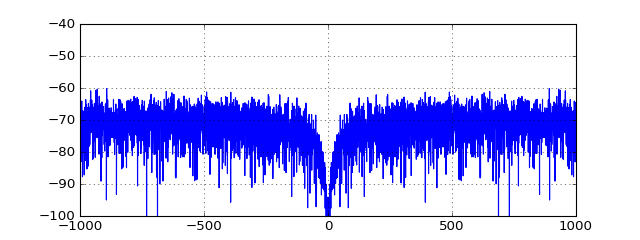Stop received from external


Then, let's plot the signal when sent over the simulated channel:

In :
runNoiseTransmission(lambda env: SimulatedChannel(env, channelEffect=HighpassChannelEffect(gain=0.2)))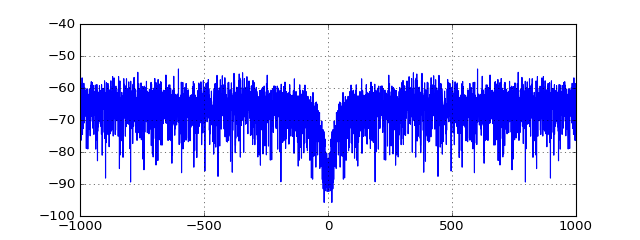Stop received from external


Looking at both spectra, it appears that the simple lowpass filter seems to quite accurately match the real sound card.

### Outlook¶

In the following notebook, we will upconvert our transmit signal to passband. In addition to the improved frequency response of the soundcard in the passband, this technique has another advantage: It allows us to move the signal into frequencies we can barely here, significantly attenuating the transmission sound. Even though it's interesting to hear the signal, it can quickly become annoying for you and your neighbors.